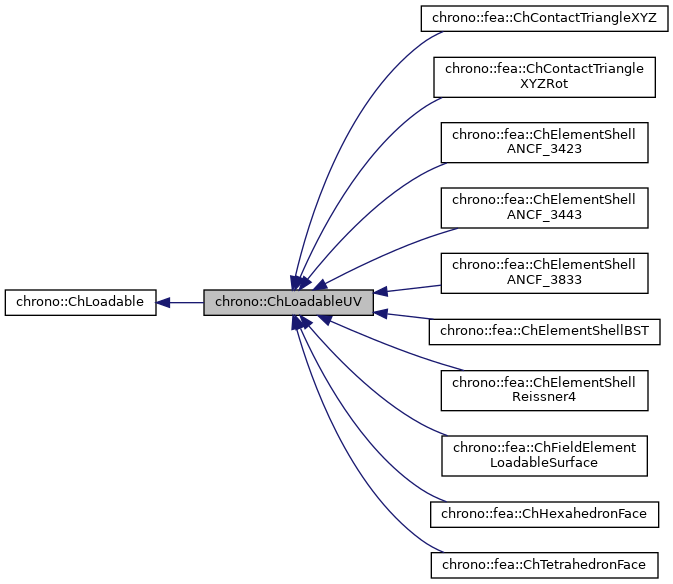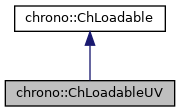## Description

Interface for objects that can be subject to area loads, distributed along UV coordinates of the object.

For instance finite elements like shells, ex.for applied pressure.

#include <ChLoadable.h>[legend][legend]

## Public Member Functions

virtual void ComputeNF (const double U, const double V, ChVectorDynamic<> &Qi, double &detJ, const ChVectorDynamic<> &F, ChVectorDynamic<> *state_x, ChVectorDynamic<> *state_w)=0
Evaluate N'*F , where N is some type of shape function evaluated at U,V coordinates of the surface, each ranging in [-1..+1], except if IsTriangleIntegrationNeeded() true. More...

virtual ChVector ComputeNormal (const double U, const double V)=0
Gets the normal to the surface at the parametric coordinate u,v. More...

virtual bool IsTriangleIntegrationNeeded ()
If true, use quadrature over u,v in [0..1] range as triangle area coords (with z=1-u-v) otherwise use default quadrature over u,v in [-1..+1] as rectangular isoparametric coords.Public Member Functions inherited from chrono::ChLoadable
Gets the number of DOFs affected by this element (position part)

Gets the number of DOFs affected by this element (speed part)

virtual void LoadableGetStateBlock_x (int block_offset, ChState &mD)=0
Gets all the DOFs packed in a single vector (position part)

virtual void LoadableGetStateBlock_w (int block_offset, ChStateDelta &mD)=0
Gets all the DOFs packed in a single vector (speed part)

virtual void LoadableStateIncrement (const unsigned int off_x, ChState &x_new, const ChState &x, const unsigned int off_v, const ChStateDelta &Dv)=0
Increment all DOFs using a delta. More...

virtual int Get_field_ncoords ()=0
Number of coordinates in the interpolated field (e.g., 3 for a tetrahedron, 1 for a thermal problem, etc.).

virtual int GetSubBlocks ()=0
Get the number of DOFs sub-blocks (e.g., 1 for a body, 4 for a tetrahedron, etc.).

virtual unsigned int GetSubBlockOffset (int nblock)=0
Get the offset of the specified sub-block of DOFs in global vector.

virtual unsigned int GetSubBlockSize (int nblock)=0
Get the size of the specified sub-block of DOFs in global vector.

virtual bool IsSubBlockActive (int nblock) const =0
Check if the specified sub-block of DOFs is active.

virtual void LoadableGetVariables (std::vector< ChVariables * > &mvars)=0
Get the pointers to the contained ChVariables, appending to the mvars vector.

## ◆ ComputeNF()

 virtual void chrono::ChLoadableUV::ComputeNF ( const double U, const double V, ChVectorDynamic<> & Qi, double & detJ, const ChVectorDynamic<> & F, ChVectorDynamic<> * state_x, ChVectorDynamic<> * state_w )
pure virtual

Evaluate N'*F , where N is some type of shape function evaluated at U,V coordinates of the surface, each ranging in [-1..+1], except if IsTriangleIntegrationNeeded() true.

F is a load, N'*F is the resulting generalized load Returns also det[J] with J=[dx/du,..], that might be useful in gauss quadrature.

Parameters
 U parametric coordinate in surface V parametric coordinate in surface Qi Return result of N'*F here detJ Return det[J] here F Input F vector, size is =n. field coords. state_x if != 0, update state (pos. part) to this, then evaluate Q state_w if != 0, update state (speed part) to this, then evaluate Q

## ◆ ComputeNormal()

 virtual ChVector chrono::ChLoadableUV::ComputeNormal ( const double U, const double V )
pure virtual

Gets the normal to the surface at the parametric coordinate u,v.

Normal must be considered pointing outside in case the surface is a boundary to a volume.

The documentation for this class was generated from the following file: